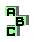POINT X(55)

 r50 tangential triangle, intangential triangle, extangential triangle, homothetic triangles r51 Gob point, Clawson point, point X(33) r149 circumcircle, incircle, tangent circles, perspective r193 Schröder point, Weill point,Bevan-Schröder point, Bevan point, poristic triangles, point X(65), point X(56) d Schröder point r457 tangent circles r546 intouch triangle, homothetic triangles r561 intouch triangle, incircle, cevian triangle, Weill point r734 antiorthic axis, circumcircle r867 intouch triangle, orthic triangle, incircle, homothetic triangles r890 tripolar, isogonal points, circumcircle, incircle, cevian quotient, Mittenpunkt r1138 circumcircle, incircle, circumcevian triangle, point X(57) r1156 point X(56), inconic, perspector, Feuerbach point, isogonal points r1176 homothetic triangles, tangent circles r1594 incenter, point X(57), point X(165) r1655 excircles, incircle, isotomic points, angent circles r1690 symmedian point, Feuerbach point, point X(37), concyclic points r1921 cevian triangle